## Monday, July 13, 2015

### More on a new method for co-phylogenetic tree plotting

Today I have continued to play around with the method I posted yesterday to create a kind of “co-phylogenetic plot” - that is, a plot of left & right facing phylograms with the tips lined up, & connected, so as to show an association between species.

The major improvements that I made are the following:

(1) I improved the tip rotation optimization method, and now allow the user to specify a function for the optimization (such as `function(x) x2`, which tells `cophylo` to minimize the sum of squares deviations between the plots; or `function(x) abs(x)`, which tells it to minimize the summed absolute values.

(2) I now permit the trees to have different numbers of tips and thus incomplete matching (some taxa in one or the other trees that have no match in the second).

(3) When no `assoc` matrix is supplied, `cophylo` now checks for any taxa with matching labels between the two trees, not just to see if all tip labels match.

(4) Now, one tip in one tree can match to multiple tips in a second (and/or vice versa).

Finally, (5) if no associations are provided (& none can be found), the function simply returns two facing trees to be plotted by `plot.cophylo`.

In the previous version of this function I was using a slightly modified version of the phytools function `minRotate` to perform the node rotation optimization. I have replaced this with a custom internal function which is much more flexible and better suited to this particular task.

Here's a demo of some of this functionality:

``````library(phytools)
source("http://www.phytools.org/cophylo/v0.2/cophylo.R")
``````

First, the basic usage, but specifying different functions to optimize node rotation. The default is `x2`.

``````tr1<-pbtree(n=26,tip.label=LETTERS)
tr2<-pbtree(n=26,tip.label=sample(LETTERS))
obj<-cophylo(tr1,tr2,rotate=FALSE)
plot(obj)
``````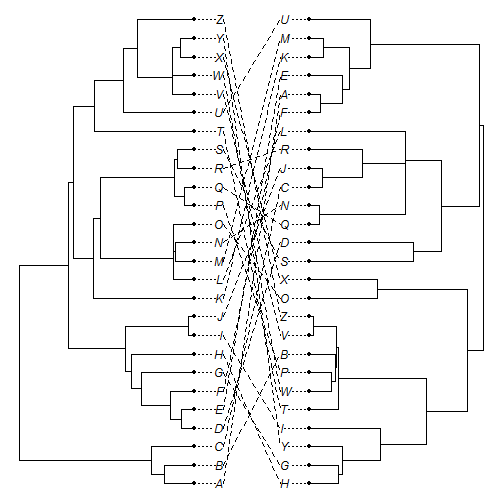``````obj<-cophylo(tr1,tr2)
``````
``````## Rotating nodes to optimize matching...
## Done.
``````
``````plot(obj)
``````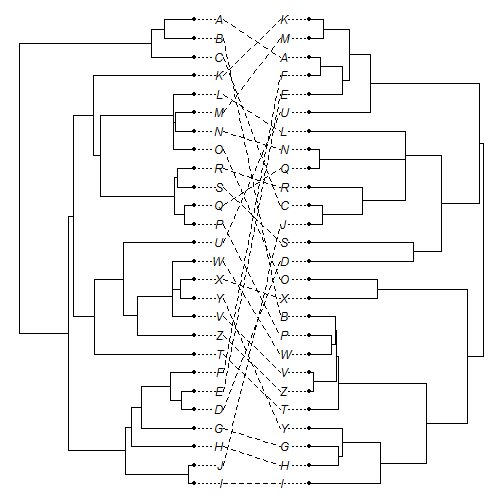``````obj<-cophylo(tr1,tr2,fn=function(x) abs(x))
``````
``````## Rotating nodes to optimize matching...
## Done.
``````
``````plot(obj)
``````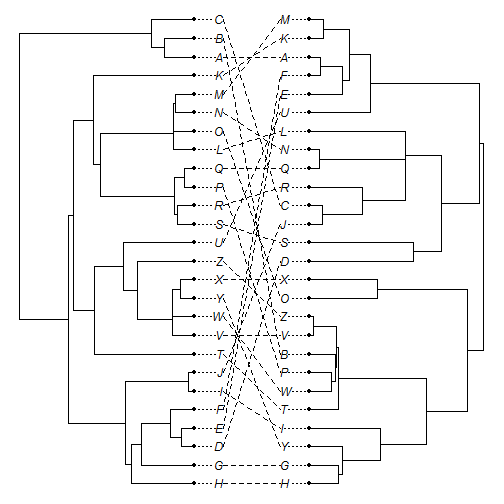Now, multiple one tip in `tr1` match to multiple tips in `tr2` and vice versa:

``````assoc<-cbind(c("A","A","A","X","Y","Z"),
c("A","B","C","Z","Z","Z"))
assoc
``````
``````##      [,1] [,2]
## [1,] "A"  "A"
## [2,] "A"  "B"
## [3,] "A"  "C"
## [4,] "X"  "Z"
## [5,] "Y"  "Z"
## [6,] "Z"  "Z"
``````
``````obj<-cophylo(tr1,tr2,assoc,rotate=FALSE)
plot(obj)
``````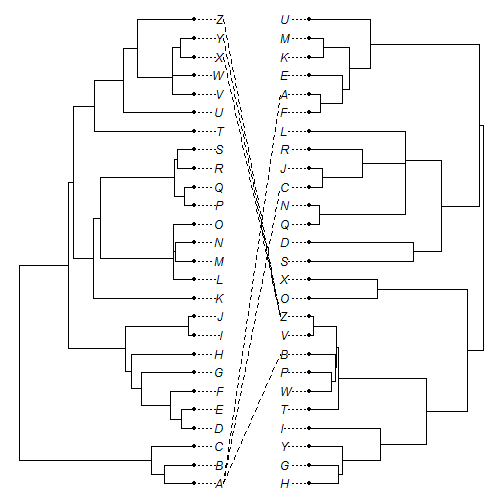``````obj<-cophylo(tr1,tr2,assoc)
``````
``````## Rotating nodes to optimize matching...
## Done.
``````
``````plot(obj)
``````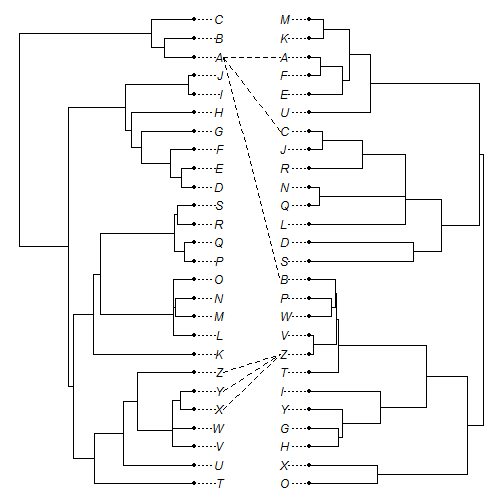Now, a partial matching of tip labels between trees:

``````tr1<-pbtree(n=12,tip.label=sample(LETTERS[1:12]))
tr2<-pbtree(n=12,tip.label=sample(LETTERS[7:18]))
obj<-cophylo(tr1,tr2)
``````
``````## Rotating nodes to optimize matching...
## Done.
``````
``````plot(obj)
``````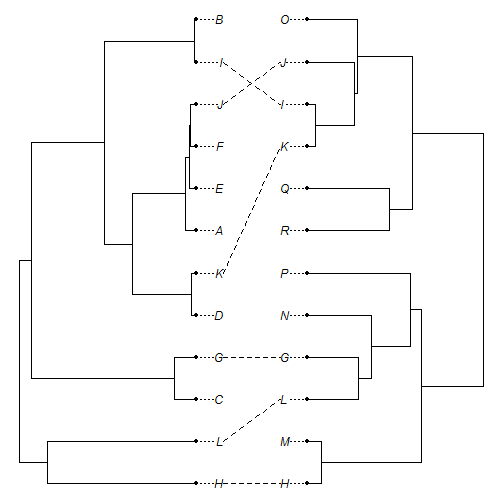Trees with different numbers of tips and partial matching:

``````tr1<-rtree(n=40)
tr2<-rtree(n=26,tip.label=LETTERS)
assoc<-cbind(sample(tr1\$tip.label,20),sample(LETTERS,20))
assoc
``````
``````##       [,1]  [,2]
##  [1,] "t25" "U"
##  [2,] "t12" "Z"
##  [3,] "t37" "A"
##  [4,] "t22" "N"
##  [5,] "t39" "H"
##  [6,] "t9"  "L"
##  [7,] "t8"  "K"
##  [8,] "t23" "D"
##  [9,] "t33" "W"
## [10,] "t18" "X"
## [11,] "t1"  "P"
## [12,] "t4"  "G"
## [13,] "t5"  "V"
## [14,] "t19" "T"
## [15,] "t27" "O"
## [16,] "t21" "B"
## [17,] "t6"  "F"
## [18,] "t13" "S"
## [19,] "t10" "R"
## [20,] "t26" "M"
``````
``````obj<-cophylo(tr1,tr2,assoc)
``````
``````## Rotating nodes to optimize matching...
## Done.
``````
``````plot(obj)
``````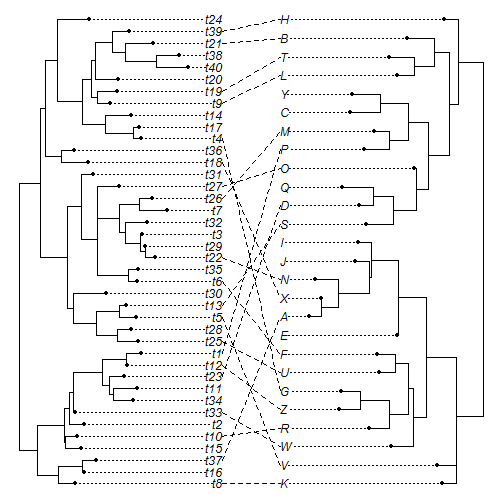Finally, no association provided (& none can be found):

``````obj<-cophylo(tr1,tr2)
``````
``````## No associations provided or found.
``````
``````plot(obj)
``````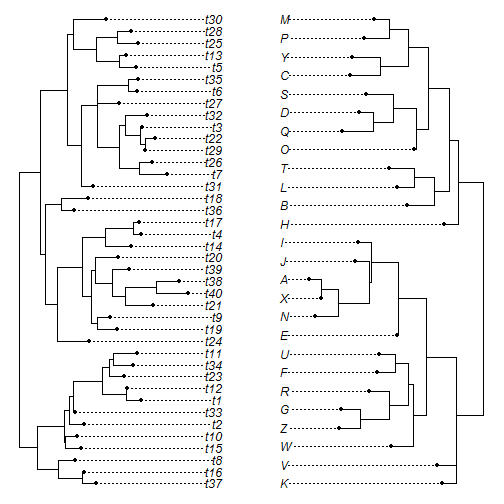``````## No associations provided.
``````

That's all for now.

1.1.This is one of the 'hidden options.' Set the argument pts to FALSE in the plot method. Let me know how that works.

2.So I uploaded two of my own trees into the R interface, what function do I use to keep my topology instead of the random letters?

1.This example used simulated trees generated using pbtree & rtree, and a random association matrix. If you delete those lines & read in your own trees instead, you should be fine.

3.This comment has been removed by the author.

4.Is it possible to change the color of the round symbols at tips and tip labels for matching metadata of the tip nodes (samples)?

1.Yes - using nodelabels.cophylo and tiplabels.cophylo: http://blog.phytools.org/2015/10/node-edge-tip-labels-for-plotted.html.

2.That's great! I will try it. Very cool! Thank you

5.Hi, This is a fantastic tool, thank you! I have two trees, one of which has less tree tips than the other and am hoping to look at the cophylogenies. However, I'm running into the following error when trying to use the plot function:

Error in xy.coords(x, y) : 'x' and 'y' lengths differ
Called from: xy.coords(x, y)

# commands:
source("http://www.phytools.org/cophylo/v0.2/cophylo.R")

# create trees

# create co-phylo object and plot
obj <-cophylo(cp26, lp54)
plot(obj)

Is there something obvious I'm doing wrong? Thank you!

6.Hi

Is it possible to use different line types (e.g. dashed via solid) with link.lty on the same plot?

Many thanks

Clive

1.This is already possible using phytools. I just posted instructions here: http://blog.phytools.org/2017/10/using-different-link-line-types-widths.html.

Note: due to the very large amount of spam, all comments are now automatically submitted for moderation.